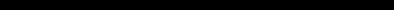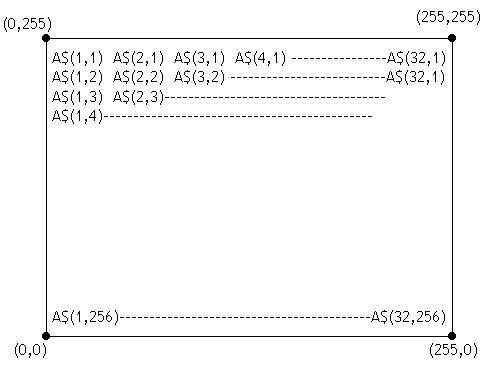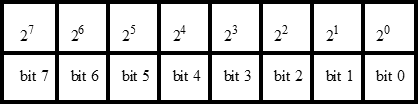# ZX PRINTER OPERATING INSTRUCTIONSProgram descriptions Lines 1 to 12 set up two distinct machine code subroutines: one at 16514 (the REM statement in line 1) the other at 31744 in reserved memory. The first simply ORs together two 8 bit numbers placed in 16526 and 16527, and allows more than one graph to be plotted on top of another. The second is the LPRINT routine, taken from the ROM and altered to use the data poked into the 256 bytes from 32256 onwards. Array A\$ takes 8k bytes of memory and contains the points to be plotted. Subroutine 9980 plots point (X,Y) into the array, and lines 9988 onwards take the finished array 256 elements at a time and hand it over to the print routine. POSITION OF ARRAY ELEMENTS ON PRINT OUTEach element of the array, e.g. A\$(1,256) contains one byte of information. A byte has eight bits which have the following values:Each bit in each byte in the array represents one dot on the high resolution printout. Hence each element of the array represent eight successive dots, the left-most dot corresponding to bit 7 whose value is 27 and the right-most dot bit 0. Hence: Dot(0,0) corresponds to bit 7 of array element A\$(1,256) Dot(1,0) corresponds to bit 6 of array element A\$(1,256) Dot(255,255) corresponds to bit 0 of array element A\$(32,1) Hence dots (0,0) through to (7,0) are represented by bits 7 through to 0 of Array Element A\$(1,256). If you wish to print all of those dots, then use the command: LET A\$(1,256)=CHR\$(2**7+2**6+2**5+2**4+2**3+2**2+2**1) If you only wish to print the first three dots then use the command: LET A\$(1,256)=CHR\$(2**7+2**6+2**5)